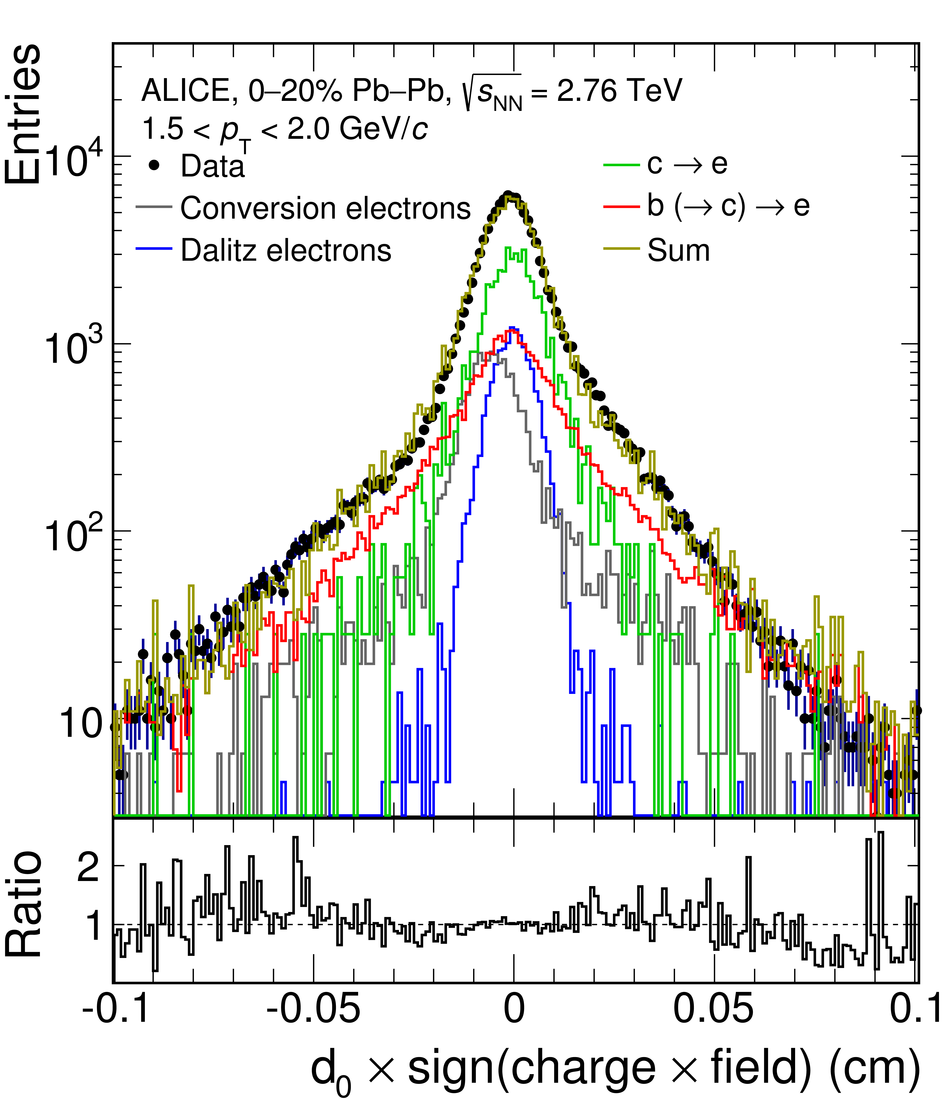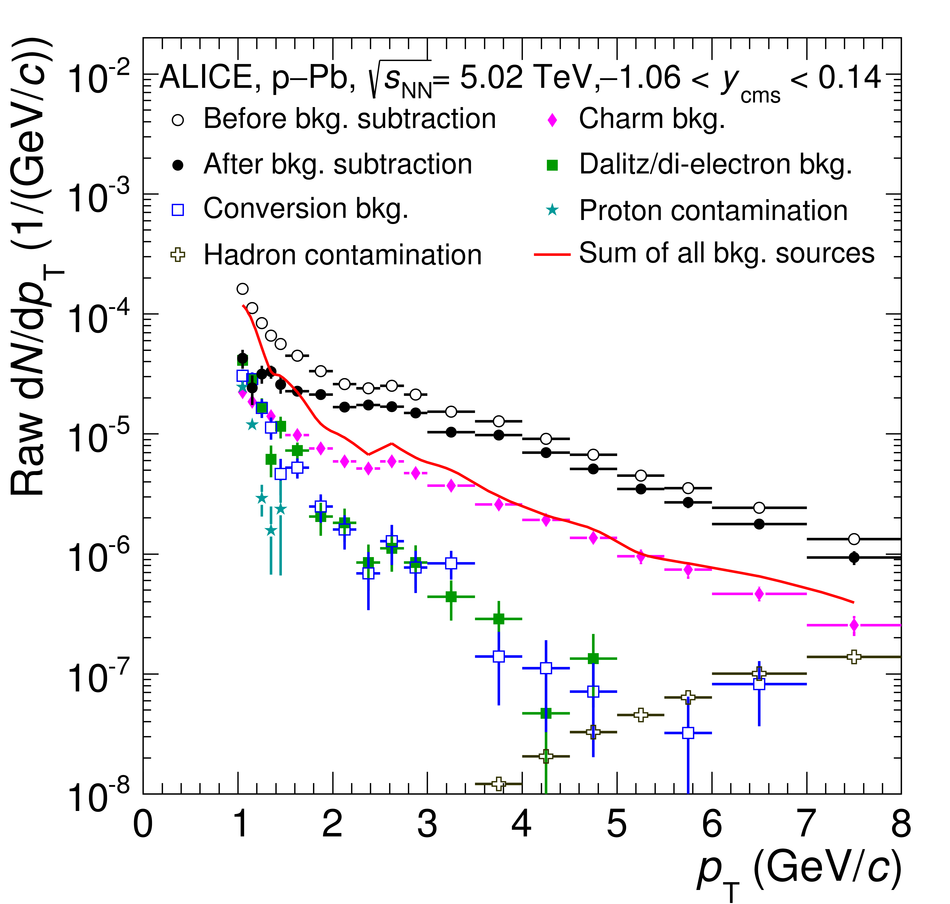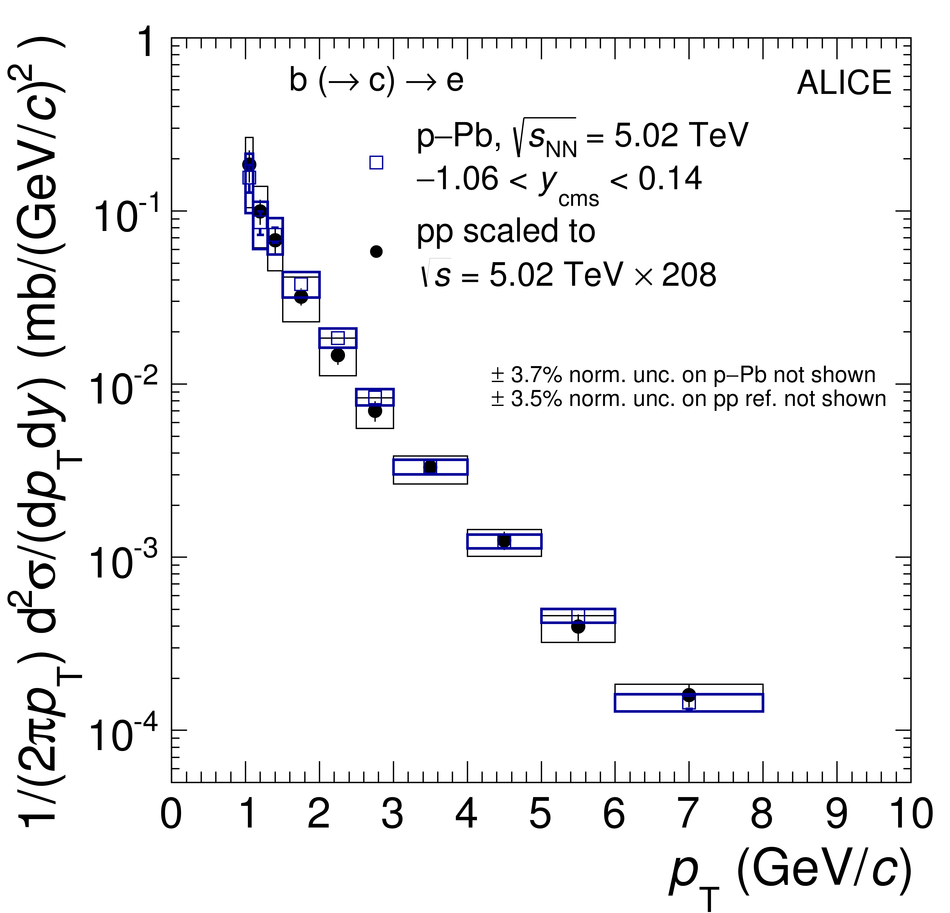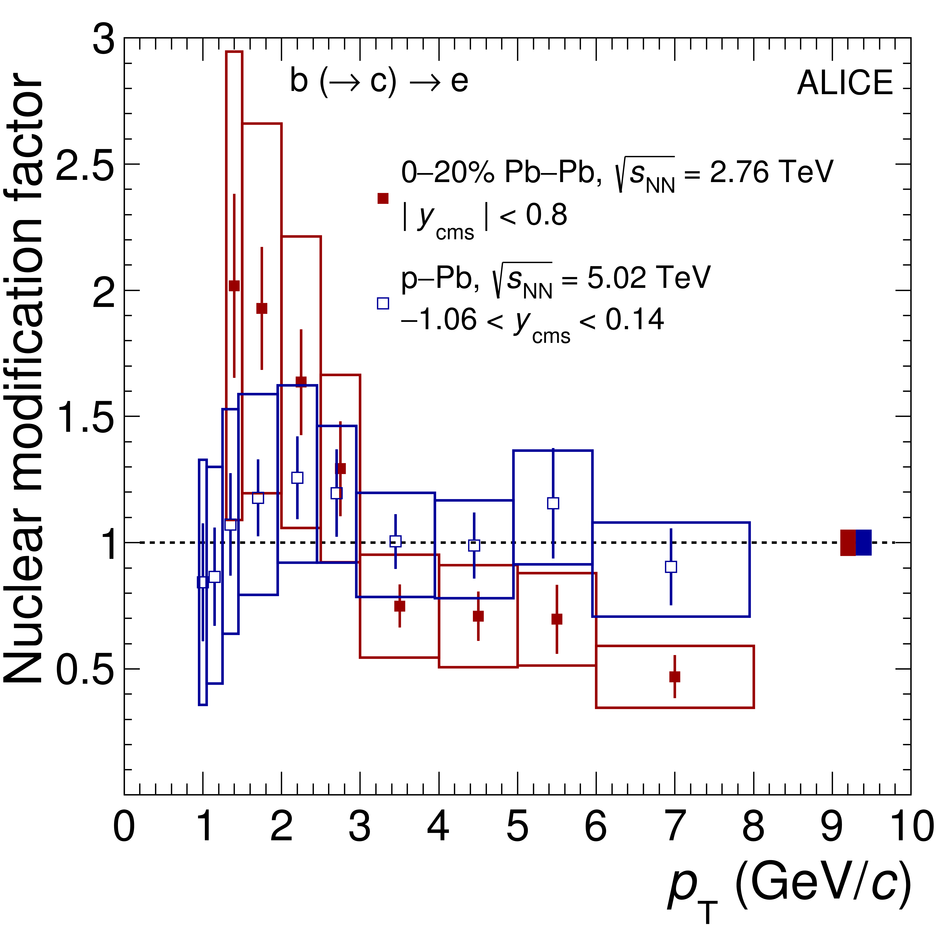# Measurement of electrons from beauty-hadron decays in p-Pb collisions at $\mathbf{\sqrt{s_{\rm NN}}=5.02}$ TeV and Pb-Pb collisions at $\mathbf{\sqrt{s_{\rm NN}}=2.76}$ TeV

The production of beauty hadrons was measured via semi-leptonic decays at mid-rapidity with the ALICE detector at the LHC in the transverse momentum interval $1<~p_{\rm T}<~8$ GeV/$c$ in minimum-bias p-Pb collisions at $\sqrt{s_{\rm NN}}=5.02$ TeV and in $1.3<~p_{\rm T}<~8$ GeV/$c$ in the 20% most central Pb-Pb collisions at $\sqrt{s_{\rm NN}}=2.76$ TeV. The pp reference spectra at $\sqrt{s}=5.02$ TeV and $\sqrt{s}=2.76$ TeV, needed for the calculation of the nuclear modification factors $R_{\rm pPb}$ and $R_{\rm PbPb}$, were obtained by a pQCD-driven scaling of the cross section of electrons from beauty-hadron decays measured at $\sqrt{s}=7$ TeV. The $R_{\rm PbPb}$ is about 0.7 with an uncertainty of about 30% in the interval $3<~p_{\rm T}<~6$ GeV/$c$ and 0.47 with an uncertainty of 25% in $6<~p_{\rm T}<~8$ GeV/$c$ in Pb-Pb collisions. Below $p_{\rm T}=3$ GeV/$c$, the $R_{\rm PbPb}$ values increase with decreasing transverse momentum with systematic uncertainties of 30-45%. The $R_{\rm pPb}$ is consistent with unity within systematic uncertainties of about 20% at high $p_{\rm T}$, increasing at low $p_{\rm T}$, and is well described by theoretical calculations that include cold nuclear matter effects in p-Pb collisions. The measured $R_{\rm pPb}$ and these calculations indicate that cold nuclear matter effects are small at high transverse momentum also in Pb-Pb collisions. Therefore, the observed reduction of $R_{\rm PbPb}$ below unity for high $p_{\rm T}$ can be ascribed to an effect of the hot and dense medium formed in Pb-Pb collisions.

Figures

## Figure 1

 Impact parameter distribution for the interval $1.5< p_{\rm T}< 2.0$ GeV/$c$ in the 20% most central Pb-Pb collisions. The impact parameter value of each track was multiplied by the sign of the charge of each track and the sign of the magnetic field. The individual distributions for electrons from beauty- and charm-hadron decays, from Dalitz-decays of light mesons, and from photon conversions were obtained by HIJING and PYTHIA simulations. The bottom panel shows the ratio of the data and Sum'.## Figure 2

 Raw transverse momentum distribution of electrons after track, eID and impact parameter requirement in comparison with the proton and hadron contamination as well as electrons from the different background sources in p-Pb collisions. The contributions of electrons from strange-hadron decays are included in the distributions labelled Dalitz/di-electron bkg.' and `Conversion bkg.'. The error bars represent the statistical uncertainties## Figure 3

 Invariant cross section of electrons from beauty-hadron decays at $\sqrt{s}=2.76$ TeV obtained by a pQCD-driven scaling of the cross section measured in pp collisions at $\sqrt{s}=7$ TeV in comparison with the measured spectrum in pp collisions at $\sqrt{s}=2.76$ TeV## Figure 4

 Invariant cross section (left) and yield (right) of electrons from beauty-hadron decays as a function of transverse momentum in minimum-bias p-Pb collisions at $\sqrt{s_{\rm NN}}=5.02$ TeV and in the 20% most central Pb-Pb collisions at $\sqrt{s_{\rm NN}}=2.76$ TeV. The pp reference spectra scaled by the number of nucleons in the Pb nucleus (A = 208) and by $\langle T_{\rm AA} \rangle$, respectively, are shown as well. The vertical bars represent the statistical uncertainties, the boxes indicate the systematic uncertainties. The pp and p-Pb normalisation uncertainties of 3.5% and 3.7% as well as the one of the nuclear overlap function $\langle T_{\rm AA} \rangle$ of 3.9% are not shown.## Figure 5

 (left) Nuclear modification factors $R_{\rm pPb}$ and $R_{\rm PbPb}$ of electrons from beauty-hadron decays at mid-rapidity as a function of transverse momentum for minimum-bias p-Pb collisions at $\sqrt{s_{\rm NN}}=5.02$ TeV and 20% most central Pb-Pb collisions at $\sqrt{s_{\rm NN}}=2.76$ TeV. The data points of the p-Pb analysis were shifted by 0.05 GeV/$c$ to the left along the $p_{\rm T}$ axis for better visibility. (right) $R_{\rm PbPb}$ of electrons from beauty-hadron decays together with the corresponding result for beauty- and charm-hadron decays for the 20% most central Pb-Pb collisions. The vertical bars represent the statistical uncertainties, while the boxes indicate the systematic uncertainties. The normalisation uncertainties, common to all points, are shown as filled boxes at high $p_{\rm T}$ for all nuclear modification factors## Figure 6

 Nuclear modification factors $R_{\rm pPb}$ (left) and $R_{\rm PbPb}$ (right) of electrons from beauty-hadron decays in comparison with different theoretical predictions, see text for details. The vertical bars represent the statistical uncertainties, while the boxes indicate the systematic uncertainties. The normalisation uncertainty, common to all points, is shown as a filled box at high $p_{\rm T}$ for both collision systems.